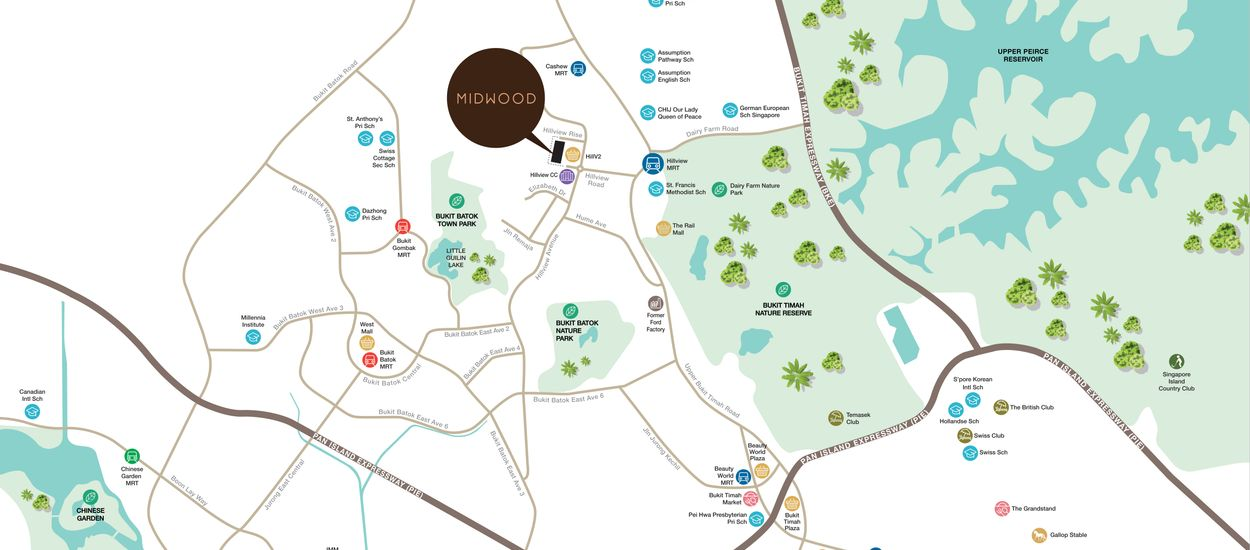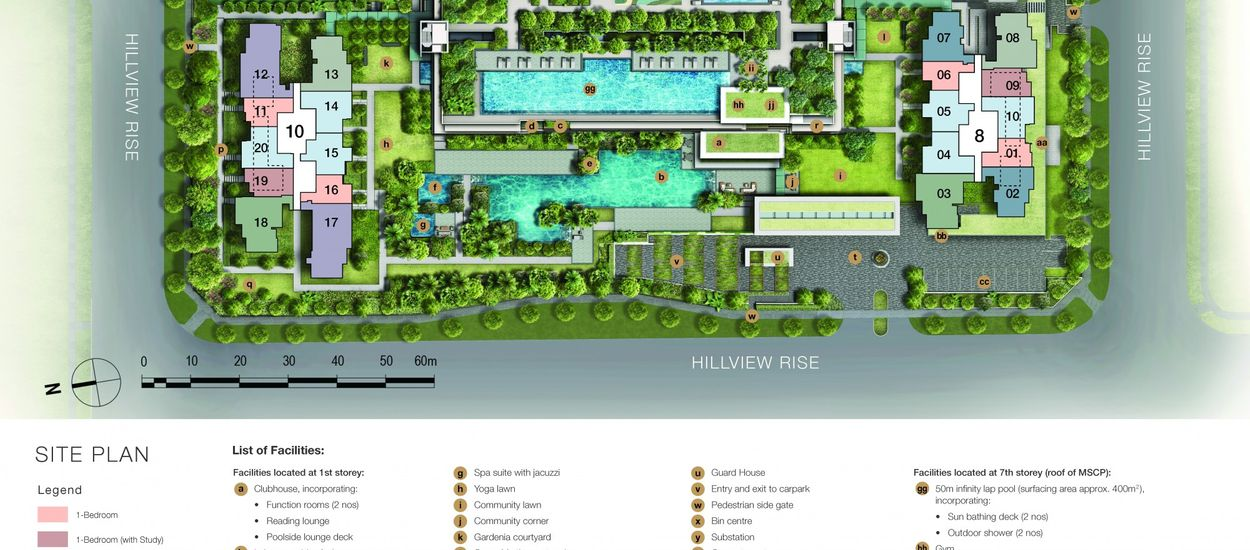### MIDWOOD

#### NON-LANDED RESIDENTIALHILLVIEW RISE,
Singapore

District: D23 - HILLVIEW, DAIRY FARM, BUKIT PANJANG, CHOA CHU KANGComplete Year

### 2024Units

### 564Property Type

### NON-LANDED RESIDENTIALTenure

### Property Details

 Project Name MIDWOOD Road HILLVIEW RISE Location Local District D23 - HILLVIEW, DAIRY FARM, BUKIT PANJANG, CHOA CHU KANG Region Central Region Broad Region Outside Central Region (OCR) Country Singapore Category NON-LANDED RESIDENTIAL Model CONDO Developer Hillview Rise Development Pte. Ltd Expected Top 1 SEP 2024 Expected Date Legal Completion 1 SEPTEMBER 2027 Tenure 99 YEARS LEASEHOLD Architect DP Architects Quantity Surveyor Davis Langdon KPK (Singapore) Pte Ltd CS Engineer KCL Consultants Pte Ltd ME Engineer UPC Pte Ltd Project Account 003-700142-2 DBS Bank Ltd Max Building Height 2 nos of 29 storey residential blocks with 6 storey MSCP Land Size Area 153,881.0 sqft Mukim Lot No 05079K MK 10 Hillview Rise Total No Units 564 units of condominium Addresses 8 Hillview Rise Singapore 667971 10 Hillview Rise Singapore 667972 Facilities Function Room Pool Deck Tennis Court Yoga Lawn Children's Playground Leisure Pool Childcare Centre Reading Room Party Deck Substation Outdoor Fitness Corner Lounge Deck Picnic Lawn Palm Court Hydrotherapy Pool Outdoor Shower Guard House Accessible Toilet/ Changing Room Gym Arrival Court Pedestrian Side Gate Generator Set Club House

### Pricing

 No. of Rooms Unit Type Sqm Sqft No. of Units Stack Price Min. Price Max. 1 Bedroom (1)b 45 484 55 01, 11 \$748,000 \$930,000 (1)b1 45 484 1 11 \$769,000 \$769,000 (1)c1 46 495 2 04, 14 \$842,000 \$842,000 (1)a 45 484 56 06, 16 \$751,000 \$985,000 (1)a1 45 484 2 06, 16 \$746,000 \$799,000 1 Bedroom (with Study) (1+1)a 51 549 55 09, 19 \$887,000 \$1,082,000 (1+1)a1 51 549 1 19 SOLD SOLD 2 Bedroom (2P)a 65 700 56 04, 14 \$1,121,000 \$1,391,000 (2P)b 64 689 56 05, 15 \$1,068,000 \$1,378,000 (2P)b1 64 689 2 05, 15 \$1,063,000 \$1,172,000 (2)a 59 635 55 10, 20 \$975,000 \$1,250,000 (2)a1 59 635 1 20 \$1,001,000 \$1,001,000 2 Bedroom (with Study) (2+1)b 72 775 27 02 \$1,279,000 \$1,482,000 (2+1)a 73 786 27 07 \$1,209,000 \$1,437,000 3 Bedroom (3)a 83 893 27 13 \$1,474,000 \$1,617,000 (3)b 84 904 27 08 \$1,396,000 \$1,594,000 3 Bedroom (with Yard) (3Y)a 92 990 28 18 \$1,529,000 \$1,755,000 (3Y)a1 92 990 1 18 \$1,555,000 \$1,555,000 (3Y)b 92 990 27 03 \$1,634,000 \$1,832,000 4 Bedroom (4)b 117 1,259 28 12 \$2,023,000 \$2,239,000 (4)b1 117 1,259 1 12 Call to enquire for pricing Call to enquire for pricing (4)a 116 1,249 28 17 \$1,928,000 \$2,144,000 (4)a1 116 1,249 1 17 \$1,960,000 \$1,960,000

### Unit

Bedroom type Area (sqft)
1 Bedroom Room 484 - 495
1 Bedroom (with Study) Room 549
2 Bedroom Room 635 - 700
2 Bedroom (with Study) Room 775 - 786
3 Bedroom Room 893 - 904
3 Bedroom (with Yard) Room 990
4 Bedroom Room 1,249 - 1,259

### Site Plan### Floor Plan

1 Bedroom

• ##### TYPE (1+1)a / (1+1)a1

1 Bedroom (with Study)

2 Bedroom

• ##### TYPE (2+1)a / (2+1)b

2 Bedroom (with Study)

3 Bedroom

• ##### TYPE (3Y)a / (3Y)a1 / (3Y)b

3 Bedroom (with Yard)

4 Bedroom

### 10 Hillview Rise

11 12 13 14 15 16 17 18 19 20
29 (1)b (4)b (3)a (2P)a (2P)b (1)a (4)a (3Y)a (1+1)a (2)a
28 (1)b (4)b (3)a (2P)a (2P)b (1)a (4)a (3Y)a (1+1)a (2)a
27 (1)b (4)b (3)a (2P)a (2P)b (1)a (4)a (3Y)a (1+1)a (2)a
26 (1)b (4)b (3)a (2P)a (2P)b (1)a (4)a (3Y)a (1+1)a (2)a
25 (1)b (4)b (3)a (2P)a (2P)b (1)a (4)a (3Y)a (1+1)a (2)a
24 (1)b (4)b (3)a (2P)a (2P)b (1)a (4)a (3Y)a (1+1)a (2)a
23 (1)b (4)b (3)a (2P)a (2P)b (1)a (4)a (3Y)a (1+1)a (2)a
22 (1)b (4)b (3)a (2P)a (2P)b (1)a (4)a (3Y)a (1+1)a (2)a
21 (1)b (4)b (3)a (2P)a (2P)b (1)a (4)a (3Y)a (1+1)a (2)a
20 (1)b (4)b (3)a (2P)a (2P)b (1)a (4)a (3Y)a (1+1)a (2)a
19 (1)b (4)b (3)a (2P)a (2P)b (1)a (4)a (3Y)a (1+1)a (2)a
18 (1)b (4)b (3)a (2P)a (2P)b (1)a (4)a (3Y)a (1+1)a (2)a
17 (1)b (4)b (3)a (2P)a (2P)b (1)a (4)a (3Y)a (1+1)a (2)a
16 (1)b (4)b (3)a (2P)a (2P)b (1)a (4)a (3Y)a (1+1)a (2)a
15 (1)b (4)b (3)a (2P)a (2P)b (1)a (4)a (3Y)a (1+1)a (2)a
14 (1)b (4)b (3)a (2P)a (2P)b (1)a (4)a (3Y)a (1+1)a (2)a
13 (1)b (4)b (3)a (2P)a (2P)b (1)a (4)a (3Y)a (1+1)a (2)a
12 (1)b (4)b (3)a (2P)a (2P)b (1)a (4)a (3Y)a (1+1)a (2)a
11 (1)b (4)b (3)a (2P)a (2P)b (1)a (4)a (3Y)a (1+1)a (2)a
10 (1)b (4)b (3)a (2P)a (2P)b (1)a (4)a (3Y)a (1+1)a (2)a
9 (1)b (4)b (3)a (2P)a (2P)b (1)a (4)a (3Y)a (1+1)a (2)a
8 (1)b (4)b (3)a (2P)a (2P)b (1)a (4)a (3Y)a (1+1)a (2)a
7 (1)b (4)b (3)a (2P)a (2P)b (1)a (4)a (3Y)a (1+1)a (2)a
6 (1)b (4)b (3)a (2P)a (2P)b (1)a (4)a (3Y)a (1+1)a (2)a
5 (1)b (4)b (3)a (2P)a (2P)b (1)a (4)a (3Y)a (1+1)a (2)a
4 (1)b (4)b (3)a (2P)a (2P)b (1)a (4)a (3Y)a (1+1)a (2)a
3 (1)b (4)b (3)a (2P)a (2P)b (1)a (4)a (3Y)a (1+1)a (2)a
2 (1)b (4)b (2P)a (2P)b (1)a (4)a (3Y)a (1+1)a (2)a
1 (1)b1 (4)b1 (1)c1 (2P)b1 (1)a1 (4)a1 (3Y)a1 (1+1)a1 (2)a1

### 8 Hillview Rise

01 02 03 04 05 06 07 08 09 10
29 (1)b (2+1)b (3Y)b (2P)a (2P)b (1)a (2+1)a (3)b (1+1)a (2)a
28 (1)b (2+1)b (3Y)b (2P)a (2P)b (1)a (2+1)a (3)b (1+1)a (2)a
27 (1)b (2+1)b (3Y)b (2P)a (2P)b (1)a (2+1)a (3)b (1+1)a (2)a
26 (1)b (2+1)b (3Y)b (2P)a (2P)b (1)a (2+1)a (3)b (1+1)a (2)a
25 (1)b (2+1)b (3Y)b (2P)a (2P)b (1)a (2+1)a (3)b (1+1)a (2)a
24 (1)b (2+1)b (3Y)b (2P)a (2P)b (1)a (2+1)a (3)b (1+1)a (2)a
23 (1)b (2+1)b (3Y)b (2P)a (2P)b (1)a (2+1)a (3)b (1+1)a (2)a
22 (1)b (2+1)b (3Y)b (2P)a (2P)b (1)a (2+1)a (3)b (1+1)a (2)a
21 (1)b (2+1)b (3Y)b (2P)a (2P)b (1)a (2+1)a (3)b (1+1)a (2)a
20 (1)b (2+1)b (3Y)b (2P)a (2P)b (1)a (2+1)a (3)b (1+1)a (2)a
19 (1)b (2+1)b (3Y)b (2P)a (2P)b (1)a (2+1)a (3)b (1+1)a (2)a
18 (1)b (2+1)b (3Y)b (2P)a (2P)b (1)a (2+1)a (3)b (1+1)a (2)a
17 (1)b (2+1)b (3Y)b (2P)a (2P)b (1)a (2+1)a (3)b (1+1)a (2)a
16 (1)b (2+1)b (3Y)b (2P)a (2P)b (1)a (2+1)a (3)b (1+1)a (2)a
15 (1)b (2+1)b (3Y)b (2P)a (2P)b (1)a (2+1)a (3)b (1+1)a (2)a
14 (1)b (2+1)b (3Y)b (2P)a (2P)b (1)a (2+1)a (3)b (1+1)a (2)a
13 (1)b (2+1)b (3Y)b (2P)a (2P)b (1)a (2+1)a (3)b (1+1)a (2)a
12 (1)b (2+1)b (3Y)b (2P)a (2P)b (1)a (2+1)a (3)b (1+1)a (2)a
11 (1)b (2+1)b (3Y)b (2P)a (2P)b (1)a (2+1)a (3)b (1+1)a (2)a
10 (1)b (2+1)b (3Y)b (2P)a (2P)b (1)a (2+1)a (3)b (1+1)a (2)a
9 (1)b (2+1)b (3Y)b (2P)a (2P)b (1)a (2+1)a (3)b (1+1)a (2)a
8 (1)b (2+1)b (3Y)b (2P)a (2P)b (1)a (2+1)a (3)b (1+1)a (2)a
7 (1)b (2+1)b (3Y)b (2P)a (2P)b (1)a (2+1)a (3)b (1+1)a (2)a
6 (1)b (2+1)b (3Y)b (2P)a (2P)b (1)a (2+1)a (3)b (1+1)a (2)a
5 (1)b (2+1)b (3Y)b (2P)a (2P)b (1)a (2+1)a (3)b (1+1)a (2)a
4 (1)b (2+1)b (3Y)b (2P)a (2P)b (1)a (2+1)a (3)b (1+1)a (2)a
3 (1)b (2+1)b (3Y)b (2P)a (2P)b (1)a (2+1)a (3)b (1+1)a (2)a
2 (2P)a (2P)b (1)a
1 (1)c1 (2P)b1 (1)a1#### ALYCIA

ASSOCIATE GROUP DISTRICT DIRECTOR

CEA NO. : R018304D